# Solving Equations of Motion for Systems of Particles with MATLAB (Part - 3) Civil Engineering (CE) Notes | EduRev

## Civil Engineering (CE) : Solving Equations of Motion for Systems of Particles with MATLAB (Part - 3) Civil Engineering (CE) Notes | EduRev

The document Solving Equations of Motion for Systems of Particles with MATLAB (Part - 3) Civil Engineering (CE) Notes | EduRev is a part of the Civil Engineering (CE) Course Introduction to Dynamics and Vibrations- Notes, Videos, MCQs.
All you need of Civil Engineering (CE) at this link: Civil Engineering (CE)

Example 2: Simple satellite orbit calculation

The figure shows satellite with mass m orbiting a planet with mass M. At time t=0 the satellite has position vector r =Ri and velocity vector v = Vj . The planet’s motion may be neglected (this is accurate as long as M>>m). Calculate and plot the orbit of the satellite.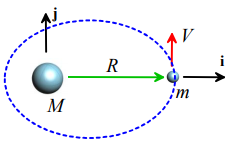1. Introduce variables to describe the motion: We will use the (x,y) coordinates of the satellite.

2. Write down the position vector in terms of these variables: r = xi + yi

3. Differentiate the position vector with respect to time to find the acceleration.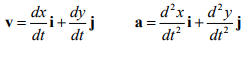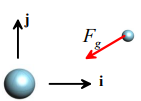4. Draw a free body diagram. The satellite is subjected to a gravitational force.

The magnitude of the force is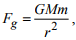where

• G is the gravitational constant, and
•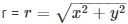is the distance between the planet and the satellite

The direction of the force is always towards the origin: -r / r is therefore a unit vector parallel to the direction of the force. The total force acting on the satellite is therefore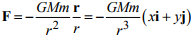5. Write down Newton’s laws of motion.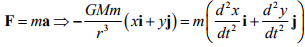The vector equation represents two separate differential equations of motion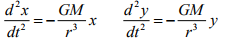6. Eliminate reactions – this is not needed in this example.

7. Identify initial conditions. The initial conditions were given in this problem - we have that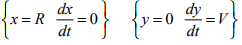8. Solve the equations of motion. We follow the usual procedure: (i) convert the equations into MATLAB form; and (ii) code a MATLAB script to solve them.

Converting the equations of motion:  We introduce the time derivatives of (x,y) as new unknown variables.   In other words, we will solve for (x, y, vx , vy), where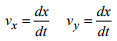These definitions are new equations of motion relating our unknown variables. In addition, we can rewrite our original equations of motion as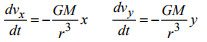So, expressed as a vector valued differential equation, our equations of motion are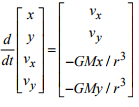Matlab script: Here’s a simple script to solve these equations.

function satellite_orbit
% Function to plot orbit of a satellite
% launched from position (R,0) with velocity (0,V)

GM=1;
R=1;
V=1;
Time=100;
w0 = [R,0,0,V]; % Initial conditions
[t_values,w_values] = ode45(@odefunc,[0,time],w0);
plot(w_values(:,1),w_values(:,2))

function dwdt = odefunc(t,w)
x=w(1); y=w(2); vx=w(3); vy=w(4);
r = sqrt(x^2+y^2);
dwdt = [vx;vy;-GM*x/r^3;-GM*y/r^3];

end

end

Running the script produces the result shown (the plot was annotated by hand)

Do we believe this result? It is a bit surprising – the satellite seems to be spiraling in towards the planet. Most satellites don’t do this – so the result is a bit suspicious. The First Law of Scientific Computing states that ` if a computer simulation predicts a result that surprises you, it is probably wrong.’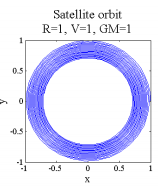So how can we test our computation? There are two good tests:

1. Look for any features in the simulation that you can predict without computation, and compare your predictions with those of the computer.
2. Try to find a special choice of system parameters for which you can derive an exact solution to your problem, and compare your result with the computer We can use both these checks here.

1. Conserved quantities For this particular problem, we know that (i) the total energy of the system should be constant; and (ii) the angular momentum of the system about the planet should be constant (these conservation laws will be discussed in the next chapter – for now, just take this as given). The total energy of the system consists of the potential energy and kinetic energy of the satellite, and can be calculated from the formula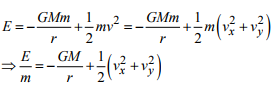The total angular momentum of the satellite (about the origin) can be calculated from the formula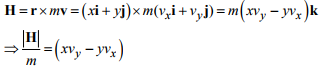(If you don’t know these formulas, don’t panic – we will discuss energy and angular momentum in the next part of the course)

We can have MATLAB plot E/m and |H| / m , and see if these are really conserved. The energy and momentum can be calculated by adding these lines to the MATLAB script

for i =1:length(t)
r = sqrt(w_values(i,1)^2 + w_values(i,2)^2)
vmag = sqrt(w_values(i,1)^2 + w_values(i,2)^2)
energy(i) = -GM/r + vmag^2/2;
angularm(i) = w_values(i,1)*w_values(i,4)-w_values(i,2)*w_values(i,3);

end

You can then plot the results (e.g. plot(t_values,energy)). The results are shown below.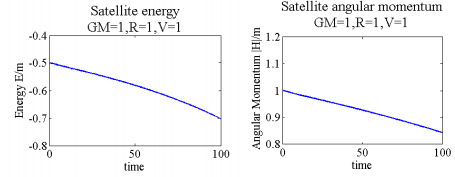These results look really bad – neither energy, nor angular momentum, are conserved in the simulation. Something is clearly very badly wrong.

Comparison to exact solution: It is not always possible to find a convenient exact solution, but in this case, we might guess that some special initial conditions could set the satellite moving on a circular path. A circular path might be simple enough to analyze by hand. So let’s assume that the path is circular, and try to find the necessary initial conditions. If you still remember the circular motion formulas, you could use them to do this. But only morons use formulas – here we will derive the solution from scratch. Note that, for a circular path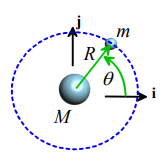(a) the particle’s radius r=constant. In fact, we know r=R, from the position at time t=0.
(b) The satellite must move at constant speed, and the angle θ must increase linearly with time, i.e. θ = ωt where θ = ω is a constant (see section 3.1.3 to review motion at constant speed around a circle).

With this information we can solve the equations of motion. Recall that the position, velocity and acceleration vectors for a particle traveling at constant speed around a circle are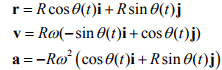We know that |v| = V from the initial conditions, and |v| is constant. This tells us that

V = Rω

Finally, we can substitute this into Newton’s law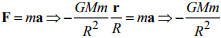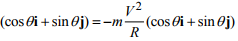Both components of the equation of motion are satisfied if we choose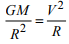So, if we choose initial values of GM V R , , satisfying this equation, the orbit will be circular. In fact, our original choice, GM = 1,V  = 1, R =1 should have given a circular orbit. It did not. Again, this means our computer generated solution is totally wrong.

Fixing the problem: In general, when computer predictions are suspect, we need to check the following

1. Is there an error in our MATLAB program? This is nearly always the cause of the problem. In this case, however, the program is correct (it’s too simple to get wrong, even for me).

2. There may be something wrong with our equations of motion (because we made a mistake in the derivation). This would not explain the discrepancy between the circular orbit we predict and the simulation, since we used the same equations in both cases.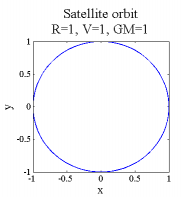3. Is the MATLAB solution sufficiently accurate? Remember that by default the ODE solver tries to give a solution that has 0.1% error. This may not be good enough. So we can try solving the problem again, but with better accuracy. We can do this by modifying the MATLAB call to the equation solver as follows

options = odeset('RelTol',0.00001);
[t_values,w_vlues] = ode45(@odefunc,[0,time],w0,options);

4. Is there some feature of the equation of motion that makes them especially difficult to solve? In this case we might have to try a different equation solver, or try a different way to set up the problem.

The figure on the right shows the orbit predicted with the better accuracy. You can see there is no longer any problem – the orbit is perfectly circular. The figures below plot the energy and angular momentum predicted by the computer.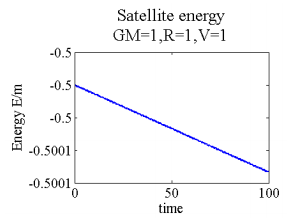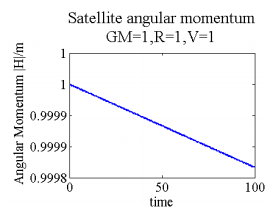There is a small change in energy and angular momentum but the rate of change has been reduced dramatically. We can make the error smaller still by using improving the tolerances further, if this is needed. But the changes in energy and angular momentum are only of order 0.01% over a large number of orbits: this would be sufficiently accurate for most practical applications.

Most ODE solvers are purposely designed to lose a small amount of energy as the simulation proceeds, because this helps to make them more stable. But in some applications this is unacceptable – for example in a molecular dynamic simulation where we are trying to predict the entropic response of a polymer, or a free vibration problem where we need to run the simulation for an extended period of time. There are special ODE solvers that will conserve energy exactly.

Example 3: Earthquake response of a 2-storey building

The figure shows a very simple idealization of a 2-storey building. The roof and second floor are idealized as blocks with mass m. They are supported by structural columns, which can be idealized as springs with stiffness k and unstretched length L. At time t=0 the floors are at rest and the columns have lengths l1 = L - mg / k l2 = L - mg / 2k (can you show this?). We will neglect the thickness of the floors themselves, to keep things simple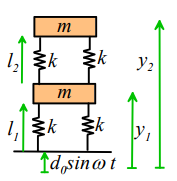For time t>0, an earthquake makes the ground vibrate vertically. The ground motion can be described using the equation d = d0 sinωt. Horizontal motion may be neglected. Our goal is to calculate the motion of the first and second floor of the building.

1. You may be skeptical that the floor of a building can be idealized as a particle (then again, maybe you couldn’t care less…). If so, you are right – it certainly is not a `small’ object. However, because the floors move vertically without rotation, the rigid body equations of motion simply reduce to F = ma and M=0, where the moments are taken about the center of mass of the block. The floors behave as though they are particles, even though they are very large.

2. Real earthquakes involve predominantly horizontal, not vertical motion of the ground. In addition, structural columns resist extensional loading much more strongly than transverse loading. So we should really be analyzing horizontal motion of the building rather than vertical motion. However, the free body diagrams for horizontal motion are messy (see if you can draw them) and the equations of motion for vertical and horizontal motion turn out to be the same, so we consider vertical motion to keep things simple

3. This problem could be solved analytically (e.g. using the `dsolve’ feature of MAPLE) – a numerical solution is not necessary. Try this for yourself.

1. Introduce variables to describe the motion: We will use the height of each floor (y1,y2) as the variables.

2. Write down the position vector in terms of these variables: We now have to worry about two masses, and must write down the position vector of both

r1 = y1 j                 r2 = y2 j

Note that we must measure the position of each mass from a fixed point.

3. Differentiate the position vector with respect to time to find the acceleration.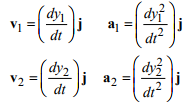4. Draw a free body diagram. We must draw a free body diagram for each mass. The resultant force acting on the bottom and top masses, respectively, are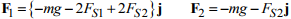where FS1, FS2 are the forces in the two springs (note that we assume that all the springs are in tension – this makes the calculation easier).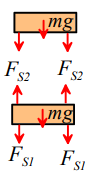The spring forces are equal to the stiffness multiplied by the increase in length of the springs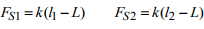We will have to find the spring lengths l1, l2 in terms of our coordinates y1,y2 to solve the problem. Geometry shows that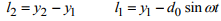So, finally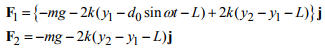5. Write down Newton’s laws of motion. F=ma for each mass gives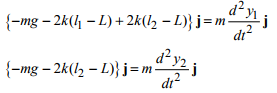This is two equations of motion – we can substitute for I1,I2  and rearrange them as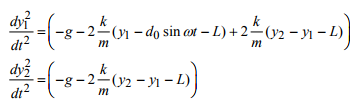6. Eliminate reactions – this is not needed in this example.

7. Identify initial conditions. We know that, at time t=0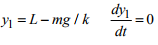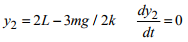8. Solve the equations of motion. We need to (i) reduce the equations to the standard MATLAB form and (ii) write a MATLAB script to solve them.

Converting the equations. We now need to do two things: (a) remove the second derivatives with respect to time, by introducing new variables; and (b) rearrange the equations into the form dy / dt = f(t,y) . We remove the derivatives by introducing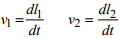as additional unknown variables, in the usual way. Our equations of motion can then be expressed as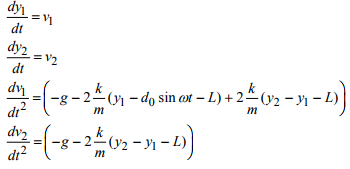We can now code MATLAB to solve these equations directly for dy/dt. A script (which plots the position of each floor as a function of time) is shown below.

function building
%
k=100;
m=1;
omega=9;
d=0.1;
L=10;
time=20;
g = 9.81;
w0 = [L-m*g/k,2*L-3*m*g/(2*k),0,0];
[t_values,w_values] = ode45(@eom,[0,time],w0);
plot(t_values,w_values(:,1:2));

function dwdt = eom(t,w)
y1=w(1);
y2=w(2);
v1=w(3);
v2=w(4);

dwdt = [v1;v2;...
-2*k*(y1-d*sin(omega*t)-L)/m+2*k*(y2-y1-L)/m;...
-g-2*k*(y2-y1-L)/m]

end

end

The figures below plot the height of each floor as a function of time, for various earthquake frequencies. For special earthquake frequencies (near the two resonant frequencies of the structure) the building vibrations are very severe. As long as the structure is designed so that its resonant frequencies are well away from the frequency of a typical earthquake, it will be safe.

We will discuss vibrations in much more detail later in this course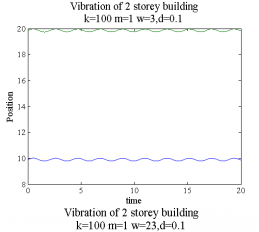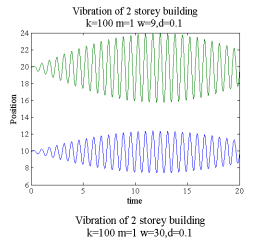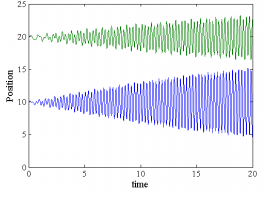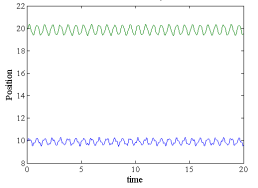This problem illustrates a shortcoming of solving problems on the computer without thinking too much about them. The way we set up the problem, it looks as though the solution depends on g, and the unstretched spring length L, but in fact this is not the case. Not being aware of this makes the equations of motion much more complicated than they really are, and makes it harder to interpret the results. Of course we could learn by trial and error that the solution is independent of L and g, but a better approach is to eliminate these variables from the equations of motion altogether.

There is a standard way to do this – instead of solving for the lengths of the springs y, we solve for the deflection of the masses from their static equilibrium positions. We will discuss this in more general terms when we discuss vibration problems later in the course. But we’ll work through the process here, because it’s useful to use the same approach in the mass launcher design project.

The figure illustrates the idea. The figure on the left shows the masses at their static equilibrium positions. Here the springs have lengths I1 = L - mg / k, l2 = L - mg / 2k. We are now going to describe the motion by the displacement of each mass from its static equilibrium position, z2, z2. We simply work through the derivations again with these new variables.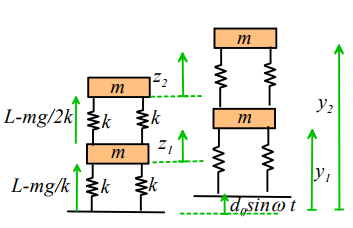The accelerations of the masses don’t depend on what we use for the origin (provided the origin is fixed, of course), so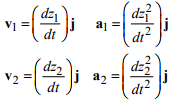The free body diagram doesn’t change either, and the forces in the springs are still given by

FS1 = k (l1 - L) FS2 = k (l2 - L)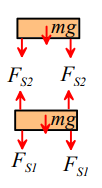But now we have to find the spring lengths  l1,l2 in terms of our coordinates z1,z2  to solve the problem. Geometry shows that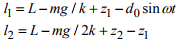Newton’s laws of motion now become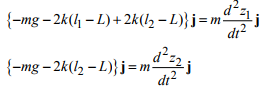Finally substituting for l1,l2 and simplifying we find that lots of terms magically cancel, and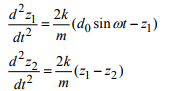These equations don’t involve L or g. Furthermore the initial conditions are simply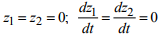so the solution for z1,z2 must be independent of L and g.

Offer running on EduRev: Apply code STAYHOME200 to get INR 200 off on our premium plan EduRev Infinity!

## Introduction to Dynamics and Vibrations- Notes, Videos, MCQs

20 videos|53 docs

,

,

,

,

,

,

,

,

,

,

,

,

,

,

,

,

,

,

,

,

,

;